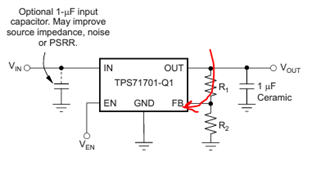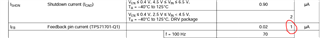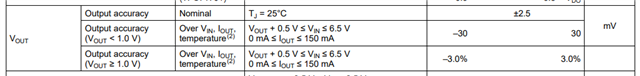If you have a related question, please click the "Ask a related question" button in the top right corner. The newly created question will be automatically linked to this question.

# TPS717: Vout tolerance due to R2 min value and Ifb max requirements

Part Number: TPS717

Hello TI,

I'm trying to continue here the thread on the min requirement for the lower feedback resistor R2 (160Kohms).

when trying to achieve relatively high output voltage e.g. 5V, the implied upper resistor R1 minimal value is calculated to be ~850KOhms:

Vout = 0.793 * (1+850/160) ~ 5.0V

When i'm conducting a worst case circuit analysis and trying to calculate the maximal output voltage, i must take into

account also the Ifb (current into the feedback pin)which can be up to 1uA according to the datasheet (with nominal value of 0.02uA ???):As far is it can be seen, to the output voltage i need to add also the voltage built on R1 due to this Ifb current:

1uA * 850Kojms = 0.85V

i.e. i assume that   Vout = Vref *(1 + (R1/R2)) + Ifb*R1 ~ 5.85V

(this before starting to include also the tolerances of Vfb, R1, R2).

This is a high, probably unacceptable, Vout max in many of the use cases.

Please advise, is there anything wrong with my assumptions, or is this the reality with this device, I need to choose between low noise or reasonable Vout max.

thanks a lot,

amotz

• Hello Amotz,

In order to calculate the worst possible case for this device, I would use the accuracy spec of this device.Meaning that the device can deviate, at 5.0V by +/-0.15V.

1uA is the amount of current that can flow into the pin before the pin is damaged an will not longer set the appropriate output value.

Best,

Juliette